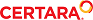﻿ Noncompartmental Analysis

NCA parameter formulas

Plasma or serum data

Data structure: NCA for blood concentration data requires the following input data:

Time of each sample

Plasma or serum concentrations

Output: Models 200–202 estimate the parameters in the following tables.

 Parameter Definition Dosing time Available as ‘Time’ in the Dosing Used results. Time of last administered dose. It is assumed to be zero unless oth­erwise specified. This parameter is used mainly with steady-state data, where time may be coded as the time elapsed since the first dose, or the elapsed time since the time of the first dose. N_Samples This parameter reports the number of non-missing observations used in the analysis of the profile (time is at or after dosing time, the observation is numeric, and the volume is positive for urine models). It does not count points inserted by engine, e.g., inserted at dosing time. Dose Amount of last administered dose. This is assumed to be zero if not specified. No_points_Lamb­da_z Number of points used in computing Lambda Z. If Lambda Z is not estimable, zero. Tlag Time of observation prior to the first observation with a measurable (non-zero) concentration. For plasma models, Tlag is only computed when the dosing type is extravascular. Tmax Time of maximum observed concentration. For non-steady-state data, the entire curve is considered. If the maximum observed concentration is not unique, then the first maximum is used. Cmax Maximum observed concentration, occurring at time Tmax, as defined above. Cmax_D =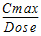C0 Initial concentration. Given only for IV Bolus dosing. It is equal to the first observed concentration value if that value occurs at the dose time. Otherwise, it is estimated by back-extrapolating (see “AUC_%Back_Ext(_obs, _pred)” below). Tlast Time of last measurable (positive) observed concentration. Clast Observed concentration corresponding to Tlast. AUClast Area under the curve from the time of dosing to the time of the last measurable (positive) concentration (Tlast). AUClast_D =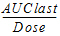AUCall Area under the curve from the time of dosing to the time of the last observation. If the last concentration is positive, AUClast=AUCall. Otherwise, AUCall will not be equal to AUClast, as it includes the additional area from the last measurable (positive) concentration down to zero or negative observations. AUMClast Area under the moment curve from the time of dosing to the last measurable (positive) concentration. MRTlast Mean residence time from the time of dosing to the time of the last measurable concentration.For non-infusion models: =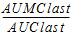For infusion models: =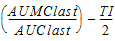where TI is the length of infusion.

The following table includes several parameters that are extrapolated to infinity. These parameters are calculated two ways: based on the last observed concentration (indicated by “_obs” appended to the parameter name), or based on the last predicted concentration (indicated by “_pred” appended to the parameter name), where the predicted value is based on the linear regression performed to esti­mate Lambda Z.

 Parameter Definition Rsq Goodness of fit statistic for the terminal elimination phase. Rsq_adjusted Goodness of fit statistic for the terminal elimination phase, adjusted for the number of points used in the estimation of Lambda Z. Corr_XY Correlation between time (X) and log concentration (Y) for the points used in the estimation of Lambda Z. Lambda_z First-order rate constant associated with the terminal (log-linear) portion of the curve. Estimated by linear regression of time vs. log concentration. Lambda_z_inter­cept Intercept on log scale estimated via linear regression of time vs. log concentration. Lambda_z_lower Lower limit on time for values to be included in the calculation of Lambda Z. Lambda_z_upper Upper limit on time for values to be included in the calculation of Lambda Z. HL_Lambda_z Terminal half-life=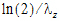Span =Clast_pred Predicted concentration at Tlast: = exp(Lambda_z_intercept – Lambda_z*Tlast) AUCINF(_obs, _pred) AUC from time of dosing extrapolated to infinity, based on the last observed concentration (_obs) or last predicted concentration (_pred).=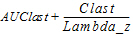AUCINF_D(_obs, _pred) =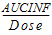AUC_%Extrap(_obs, _pred) Percentage of AUCINF(_obs, _pred) due to extrapolation from Tlast to infinity: =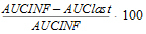AUC_%Back_Ext(_obs, _pred) Computed for IV Bolus models. Percentage of AUCINF that was due to back extrapolation to estimate C0 when the first measured concentration is not at dosing time. Vz(_obs, _pred) Vz_F(_obs, _pred)a Volume of distribution based on the terminal phase. For non-steady-state data:=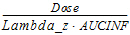Cl(_obs, _pred) Cl_F(_obs, _pred)a Total body clearance for extravascular administration.=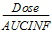AUMCINF(_obs, _pred) Area under the first moment curve (AUMC) extrapolated to infinity, based on the last observed concentration (obs) or the last predicted concentration (pred).=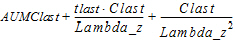AUMC_%Extrap(_obs, _pred) Percent of AUMCINF(_obs, _pred) that is extrapolated.=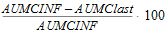MRTINF(_obs, _pred) Mean residence time (MRT) extrapolated to infinity. For non-steady-state data:For non-infusion models:=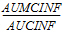For infusion models:=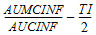where TI is the length of infusion. (Note that, for extravascular dosing (oral model 200), MRTINF includes Mean Input Time as well as time in systemic circulation.) Vss(_obs, _pred) For non-steady-state data: An estimate of the volume of distribution at steady-state based on the last observed (obs) or last predicted (pred) concentration. =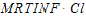Computed for IV Bolus and Infusion dosing only. Not computed for extravascular dosing (oral model 200), as MRTINF for oral models includes Mean Input Time as well as time in systemic circulation and therefore is not appropriate to use in cal­culating Vss.
 aFor extravascular models (model 200), the fraction of dose absorbed cannot be estimated; therefore Volume and Clearance for these models are actually Volume/F or Clearance/F where F is the fraction of dose absorbed.

 Parameter Definition Tau Available in the Dosing Used results worksheet for steady-state data. The (assumed equal) dosing interval for steady-state data. Tmax Time of maximum observed concentration. For steady-state data, based on observations collected during the dosing interval, that is, at or after the dosing time, but no later than the dosing time plus Tau, where Tau is the dosing interval. If the maximum observed concentration is not unique, then the first maximum is used. Cmax Maximum observed concentration, occurring at time Tmax, as defined above. Tmin Time of minimum observed concentration. For steady-state data, based on observations collected during the dosing interval (i.e., after the dosing time, but no later than dosing time plus Tau, where Tau is the dosing interval). If the minimum observed concentration is not unique, then the first minimum is used. Cmin Minimum observed concentration occurring at time Tmin as defined above. Note: Regulatory agencies differ on the definition of Cmin: some agencies define Cmin the same as Ctau is defined below. Both Cmin and Ctau are included in the output so that users can use the correct parameter for their situation. Ctau Concentration at dosing time plus Tau. Observed concentration if the value exists in the input data; other­wise, the predicted concentration value. Predicted concentrations are calculated following the same rules as for computing inserting missing endpoints needed for partial areas, see “Partial area calculation”. Cavg Average concentration, computed: =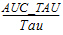Swing =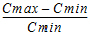Swing_Tau =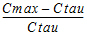Fluctuation% =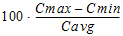where Cmin and Cmax were obtained between dosing time and dosing time plus Tau. Fluctuation%_Tau =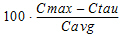CLss, CLss_Fa An estimate of the total body clearance, computed for IV Bolus and Infusion dosing only.=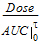MRTINF(_obs, _pred) Mean residence time (MRT) extrapolated to infinity based on AUC­INF(_obs, _pred).For non-infusion: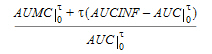For infusion: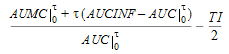where TI represents infusion duration. (Note that, for oral model 200, MRTINF includes Mean Input Time as well as time in systemic circulation.) Vz, Vz_Fa =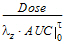Vss(_obs, _pred) An estimate of the volume of distribution at steady-state based on the last observed (obs) or last predicted (pred) concentration. Computed for IV Bolus and infusion dosing only.=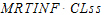Not computed for extravascular dosing (oral model 200), as MRTINF for oral models includes Mean Input Time as well as time in systemic circulation and therefore is not appropriate to use in cal­culating Vss. Accumulation Index =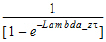AUC_TAU The partial area from dosing time to dosing time plus Tau. See “Partial area calculation” for information on how it is computed. AUC_TAU_D =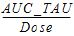AUC_TAU_%Extrap Percentage of AUC_TAU that is due to extrapolation from Tlast to infinity. =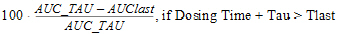=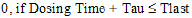AUMC_TAU Area under the first moment curve from dosing time to dosing time plus Tau. See “Partial area calculation” for information on how it is computed. AUClower_upper (Optional) User-requested area(s) under the curve from time “lower” to “upper”.
 aFor extravascular models (model 200), the fraction of dose absorbed cannot be estimated; therefore Volume and Clearance for these models are actually Volume/F or Clearance/F where F is the fraction of dose absorbed.

Urine data

Data structure: NCA for urine data requires the following input data:

Starting and ending time of each urine collection interval

Urine concentrations

Urine volumes

From this data, models 210–212 compute the following for the analysis:

Midpoint of each collection interval=(Starting time+Ending time)/2

Excretion rate for each interval (amount eliminated per unit of time)=(Concentration*Volume)/(Ending time-Starting time)

Output: Models 210–212 estimate the following parameters.

The worksheet will include the Sort(s), Carry(ies), parameter names, units, and computed values. A User Defined Parameters Pivoted worksheet will include the pivoted form of the User Defined Param­eters worksheet.

 Parameter Definition Dosing time Available as ‘Time’ in the Dosing Used results. Time of last administered dose. It is assumed to be zero unless oth­erwise specified. This parameter is used mainly with steady-state data, where time may be coded as the time elapsed since the first dose, or the elapsed time since the time of the first dose. Dose Amount of last administered dose. This is assumed to be zero if not specified. N_Samples Number of non-missing observations in the analysis. Does not include missing or non-numeric observations, observa­tions before dosing time, or urine observations where volume is zero. Does not include the point at dosing time if it was not observed but was inserted by the engine. No_points_lamb­da_z Number of points used in the computation of Lambda Z. If Lambda Z cannot be estimated, this is set to zero. Tlag Midpoint of the collection interval prior to the first collection interval with measurable (non-zero) rate. Computed for all urine models. Tmax_Rate Midpoint of the collection interval associated with the maximum observed excretion rate. If the maximum observed excretion rate is not unique, then the first maximum is used. Max_Rate Maximum observed excretion rate, at time Tmax_Rate as defined above. Mid_Pt_last Midpoint of collection interval associated with last measurable (pos­itive) observed excretion rate. Rate_last Last observed measurable (positive) rate at time Mid_Pt_last. AURC_last Area under the urinary excretion rate curve from time of dosing to Mid_Pt_last. AURC_last_D =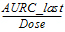Vol_UR Sum of Urine Volumes (urine) Amount_Recov­ered Cumulative amount eliminated. =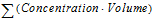Percent_Recov­ered =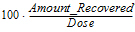AURC_all Area under the urinary excretion rate curve from the time of dosing to the midpoint of the interval with the last rate. If the last rate is positive, AURC_last=AURC_all.

The following table includes some parameters that are extrapolated to infinity. These parameters are calculated two ways: based on the last observed excretion rate: Rate_last (indicated by “_obs” appended to the parameter name), or based on the last predicted excretion rate: Rate_last_pred (indicated by “_pred” appended to the parameter name), where the predicted value is based on the linear regression performed to estimate Lambda Z.

 Parameter Definition Rsq Goodness of fit statistic for the terminal elimination phase. Rsq_adjusted Goodness of fit statistic for the terminal elimination phase, adjusted for the number of points used in the estimation of Lambda Z. Corr_XY Correlation between midpoints and log excretion rates for the points used in the estimation of Lambda Z. Lambda_z First-order rate constant associated with the terminal (log-linear) portion of the curve. This is estimated via linear regression of midpoints vs. log excretion rates. Lambda_z_inter­cept Intercept on log scale estimated via linear regression of midpoints vs. log excretion rates. Lambda_z_lower Lower limit on midpoint for values to be included in Lambda Z esti­mation. Lambda_z_upper Upper limit on midpoint for values to be included in Lambda Z esti­mation. HL_Lambda_z Terminal half-life=ln(2)/Lambda Z Span =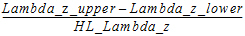Rate_last_pred Predicted rate at Mid_Pt_last. AURC_INF(_obs, _pred) Area under the urinary excretion rate curve extrapolated to infinity, based on the last observed excretion rate (_obs) or the last pre­dicted rate (_pred), i.e., the excretion rate at the final midpoint esti­mated using the linear regression for Lambda Z. Note that AURC_INF is theoretically equal to Amount_Recovered, but will differ due to experimental error. AURC_%Extrap(_obs, _pred) Percent of AURC_INF(_obs, _pred) that is extrapolated.

Sparse sampling (pre-clinical) data

When an NCA model is loaded with the Sparse Sampling option (see “Options tab”), the data are treated as a special case of plasma or urine concentration data. The NCA engine computes the mean concentration or rate at each unique time value or interval. Using the mean concentration curve across subjects, it estimates the same parameters normally calculated for plasma or urine data, plus those listed below. See “Sparse sampling calculation” for additional details of NCA computations with sparse data.

Note:The names of some of the output worksheets change when the data is sparse: Final Parameters becomes Mean Curve Final Parameters, and Summary Table becomes Mean Curve Summary Table.

Plasma or serum concentration parameters

Sparse sampling methods for plasma data (models 200–202) compute the following parameters in addition to those listed in “Plasma or serum data”.

 Parameter Definition SE_Cmax Standard error of data at Tmax (time of maximum mean concentration). SE_AUClast It is the standard error of the area under the mean concen­tration curve from dose time to Tlast, where Tlast is the time of last measurable (positive) mean concentration. SE_AUCall Standard error of the area under the mean concentration curve from dose time to the final observation time.

Note:SE_AUClast and SE_AUCall provide a measurement of the uncertainty for AUClast and AUCall, respectively, and are usually the same. Differences between these parameter values will only be observed if some of the measurements were flagged as BQL (below the quantitation limit).

Urine excretion rate parameters

Sparse sampling methods for urine data (models 210–212) compute the following parameters in addi­tion to those listed under “Urine data”.

 Parameter Definition SE_Max_Rate Standard error of the data at the time of maximum mean rate. SE_AURC_last Standard error of the area under the mean urinary excretion rate curve from dose time through the last interval that has a measurable (positive) mean rate. SE_AURC_all Standard error of the area under the mean urinary excretion rate curve from dose time through the final interval.

Individuals by time

This sheet includes the individual subject data, along with N (number of non-missing observations), the mean and standard error for each unique time value (plasma data) or unique midpoint value (urine data).

References

Holder (2001). Comments on Nedelman and Jia's extension of Satterthwaite's approximation applied to pharmacokinetics. J Biopharm Stat 11(1-2):75–9.

Nedelman, Gibiansky and Lau (1995). Applying Bailer's method for AUC intervals to sparse sampling. Pharm Res 12:124–8.

Nedelman and Jia (1998). An extension of Satterthwaite's approximation applied to pharmacokinet­ics. J Biopharm Stat 8(2):317–28.

Yeh (1990). Estimation and Significant Tests of Area Under the Curve Derived from Incomplete Blood Sampling. ASA Proceedings of the Biopharmaceutical Section 74–81.

Drug effect data model 220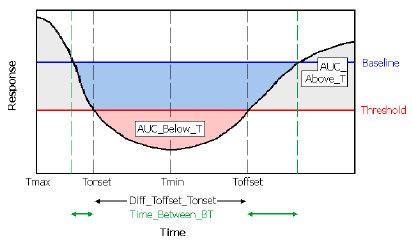Illustration of a time-effect curve with AUCs highlighted

Output parameter names use the following conventions:

B is the baseline effect value (discussed above).

T is the user-supplied threshold effect value.

“Above” means towards increasing Y values, even for inhibitory effects.

 Parameter Definition N_Samples This parameter reports the number of non-missing observations used in the analysis of the profile (time is at or after dosing time, the observation is numeric, and the volume is positive for urine models). It does not count points inserted by engine, e.g., inserted at dosing time. Slope1 (or 2) Slope of the first (or second) segment of the curve. See “Lambda Z or Slope Estimation settings”. Rsq_Slope1 (or 2) Goodness of fit statistic for slope 1 or 2. Rsq_adj_Slope1 (or 2) Goodness of fit statistic for slope 1 or 2, adjusted for the number of points used in the estimation. Corr_XY_Slope1 (or 2) Correlation between time (X) and effect (or log effect, for log regres­sion) (Y) for the points used in the slope estimation. No_points_Slope1 (or 2) The number of data points included in calculation of slope 1 or 2. Slope1_lower or Slope2_lower Lower limit on Time for values to be included in the slope calcula­tion. Slope1_upper or Slope2_upper Upper limit on Time for values to be included in the slope calcula­tion. Tmin Time of minimum observed response value (Rmin). Rmin Minimum observed response value. Tmax Time of maximum observed response value (Rmax). Rmax Maximum observed response value. Baseline Baseline response (Y) value supplied by the user, (assumed to be zero if none is supplied) or, for Certara Integral studies with no user-supplied baseline value, effect value at dose time. AUC_Above_B Area under the response curve that is above the baseline (dark gray areas in the above diagram). AUC_Below_B Area that is below the baseline and above the response curve (com­bined blue and pink areas in the above diagram). AUC_Net_B = AUC_Above_B – AUC_Below_B This is likely to be a negative value for inhibitory effects. Time_Above_B Total time that Response >= Baseline. Time_Below_B Total time that Response < Baseline. Time_%Below_B = 100*Time_Below_B/(Tfinal – Tdose)where Tfinal is the final observation time and Tdose is dosing time.

When a threshold value is provided, model 220 also computes the following.

 Parameter Definition Threshold Threshold value used. AUC_Above_T Area under the response curve that is above the threshold value (combined light and dark gray areas in the above diagram). AUC_Below_T Area that is below the threshold and above the response curve (pink area in the above diagram). AUC_Net_T = AUC_Above_T – AUC_Below_T Time_Above_T Total time that Response >= Threshold. Time_Below_T Total time that Response < Threshold. Time_%Below_T = 100*Time_Below_T/(Tlast – Tdose) where Tlast is the final observation time and Tdose is dosing time. Tonset Time that the response first crosses the threshold coming from the direction of the baseline value, as shown in the above diagram. Tonset=Tdose if the first response value is across the threshold, rel­ative to baseline. The time will be interpolated using the calculation method selected in the model options. (See “Options tab”.)a Toffset Time greater than Tonset at which the curve first crosses back to the baseline side of threshold, as shown in the diagram above.a Diff_Toffset_Tonset = Toffset – Tonset Time_Between_BT Total time spent between baseline and threshold (sum of length of green arrows in diagram). AUClower_upper (Optional) user-requested area(s) under the curve from time “lower” to time “upper”.
 aUse caution in interpreting Tonset and Toffset for noisy data if Baseline and Threshold are close together.

User defined parameters

Any user defined parameters will be computed and reported in the User Defined Parameters and User Defined Parameters Pivoted worksheets. The worksheets include entries for Sort, Carry, param­eter name, unit, and estimated value. A User Defined Parameters Pivoted worksheet will include the pivoted form of the User Defined Parameters worksheet.

 Parameter Definition C

References

Holder (2001). “Comments on Nedelman and Jia's extension of Satterthwaite's approximation applied to pharmacokinetics.” J Biopharm Stat 11(1–2):75–9.

Nedelman and Jia (1998). “An extension of Satterthwaite's approximation applied to pharmacokinet­ics.” J Biopharm Stat 8(2):317–28.